# Volumes of Pyramids and Cones Geometry 10 6

• Slides: 46Volumes of Pyramids and Cones Geometry 10 -6Review• The volume of a cube is the cube of the length of its side, or V=s 3 Volume of a cube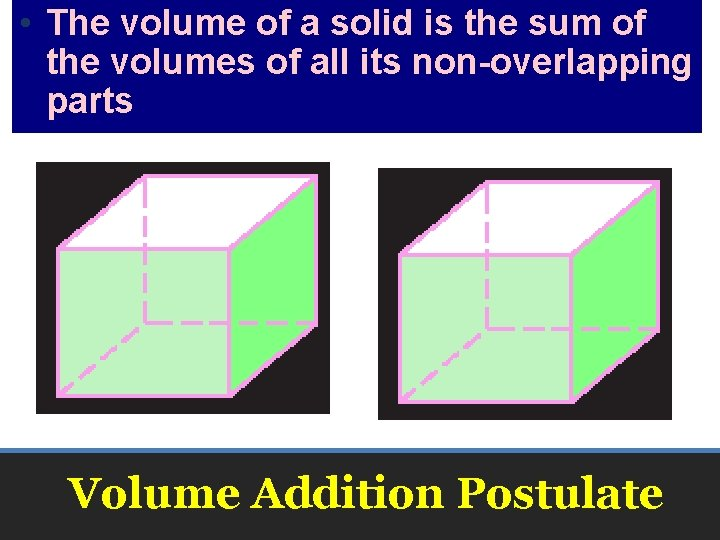• The volume of a solid is the sum of the volumes of all its non-overlapping parts Volume Addition Postulate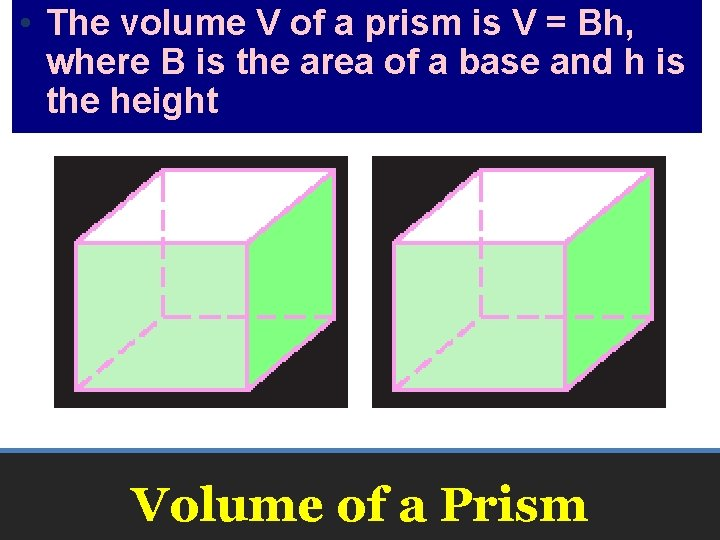• The volume V of a prism is V = Bh, where B is the area of a base and h is the height Volume of a Prism• The volume V of a cylinder is V = Bh = πr 2 h where B is the area of a base, h is the height, and r is the radius of a base Volume of a Cylinder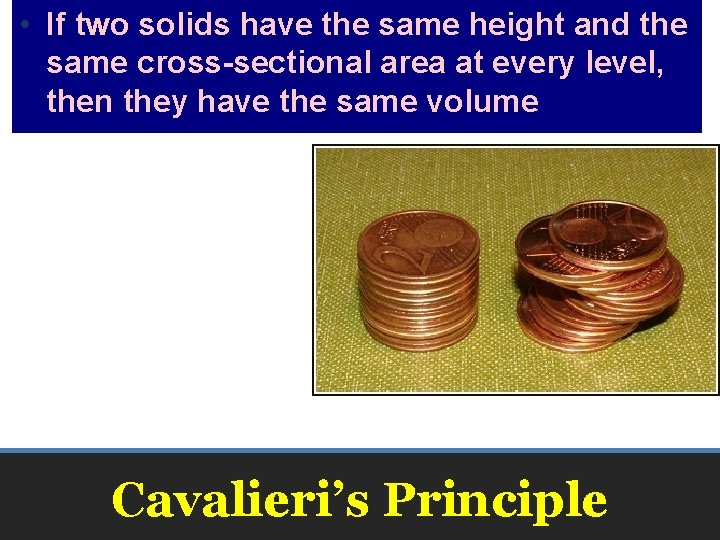• If two solids have the same height and the same cross-sectional area at every level, then they have the same volume Cavalieri’s PrincipleNew Material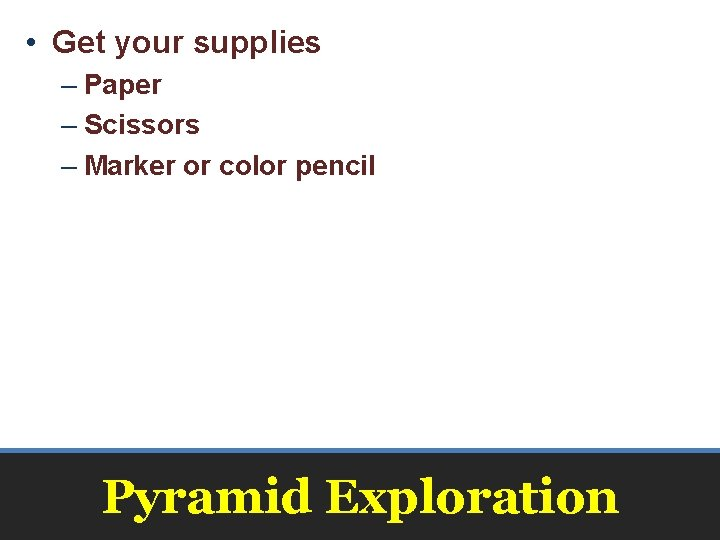• Get your supplies – Paper – Scissors – Marker or color pencil Pyramid Exploration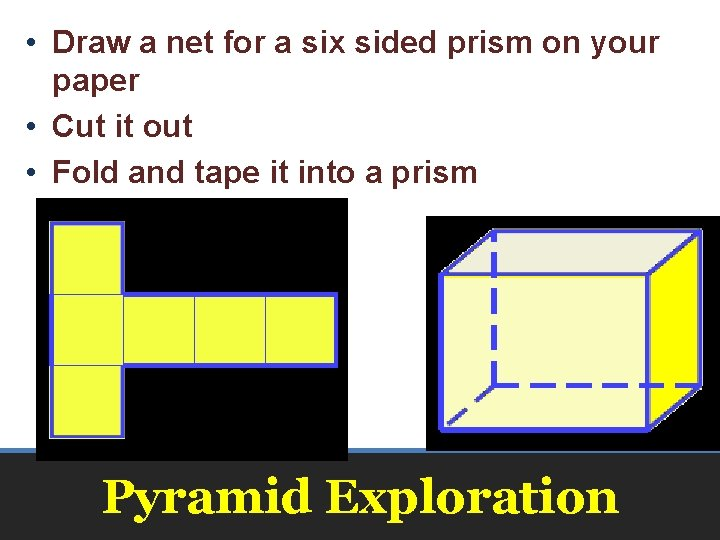• Draw a net for a six sided prism on your paper • Cut it out • Fold and tape it into a prism Pyramid Exploration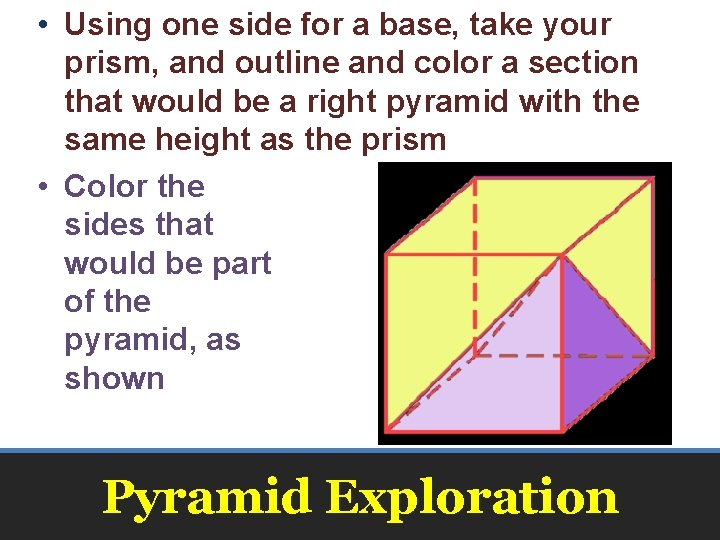• Using one side for a base, take your prism, and outline and color a section that would be a right pyramid with the same height as the prism • Color the sides that would be part of the pyramid, as shown Pyramid Exploration• Using an uncolored side as a base, make another right pyramid with the same height as the prism • Color the sides that would be part of the pyramid, a different color Pyramid Exploration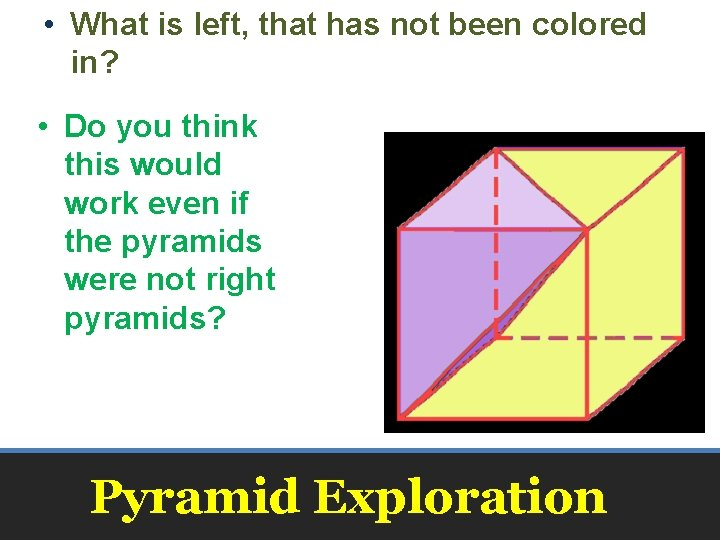• What is left, that has not been colored in? • Do you think this would work even if the pyramids were not right pyramids? Pyramid Exploration• Would this work even if the base of the pyramid was not a rectangle? Pyramid Exploration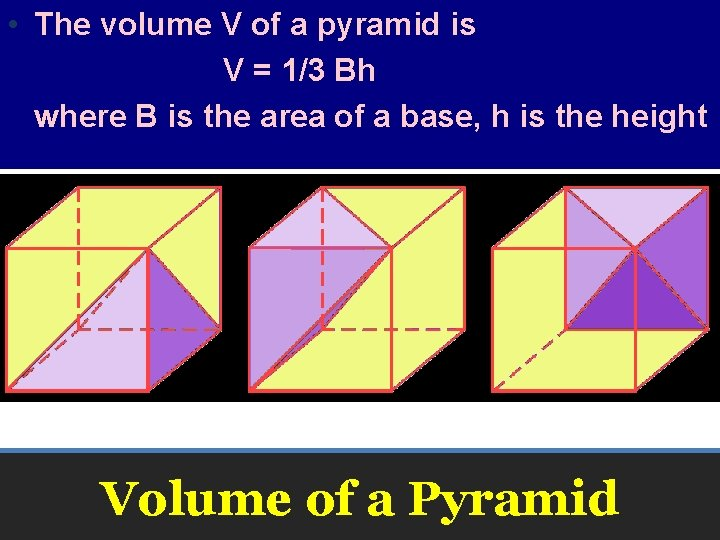• The volume V of a pyramid is V = 1/3 Bh where B is the area of a base, h is the height Volume of a Pyramid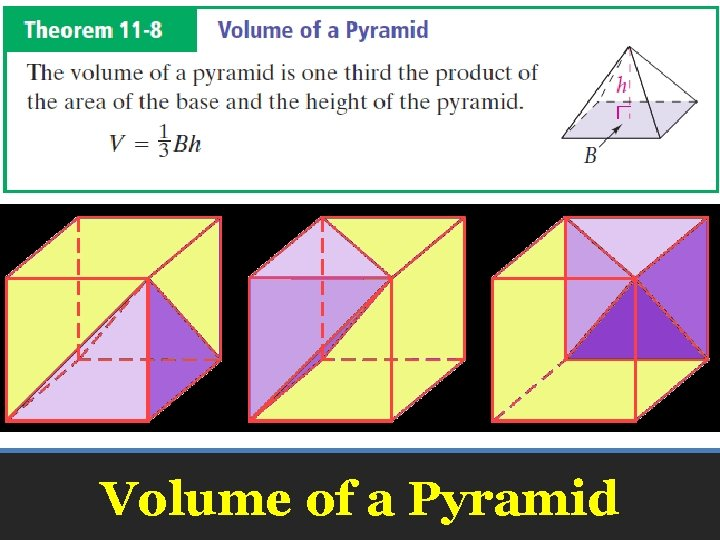• The volume V of a pyramid is V = 1/3 Bh where B is the area of a base, h is the height Volume of a Pyramid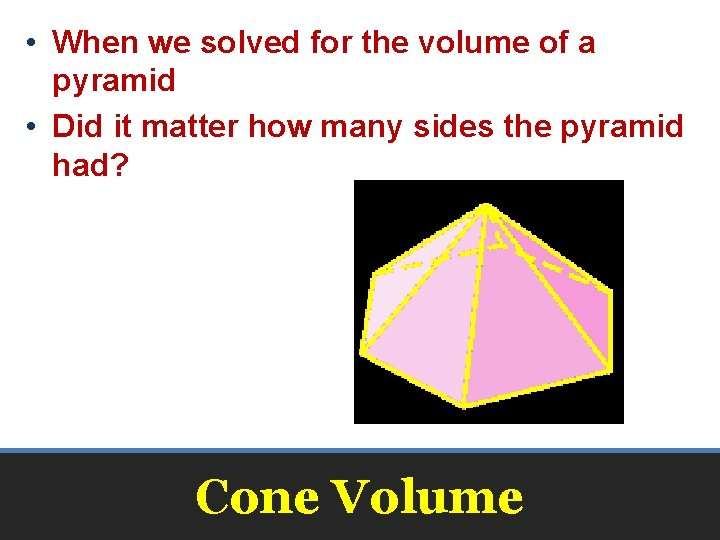• When we solved for the volume of a pyramid • Did it matter how many sides the pyramid had? Cone Volume• What if we kept increasing the number of sides of the pyramid, and the corresponding prism • what shapes do they become? Cone Volume• The volume V of a cone is V = 1/3 Bh V = 1/3 πr 2 h where B is the area of a base, h is the height and r is the radius of the cone Volume of a Cone• The volume V of a cone is V = 1/3 Bh V = 1/3 πr 2 h where B is the area of a base, h is the height and r is the radius of the cone Volume of a ConeExampleExample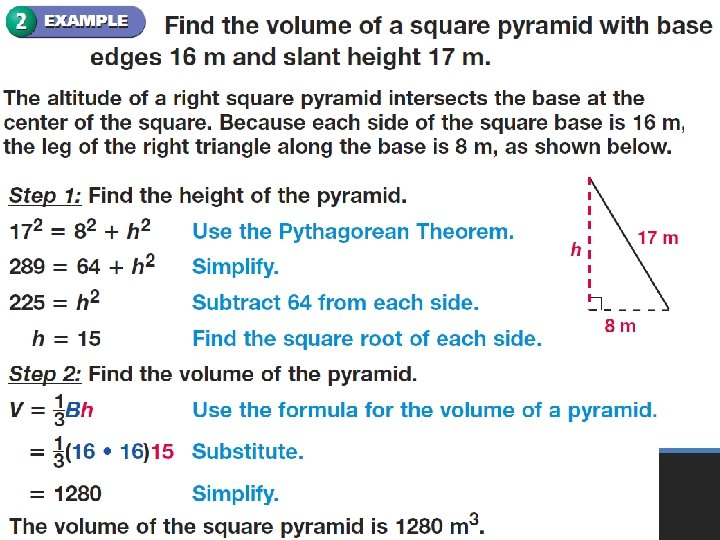Example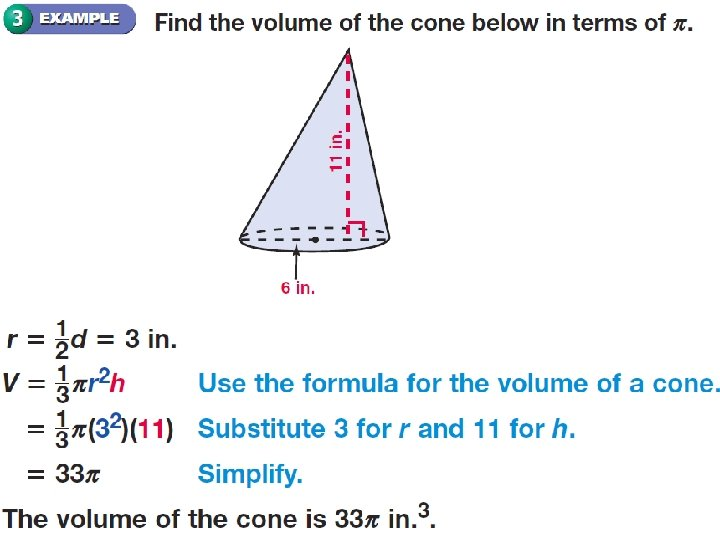Example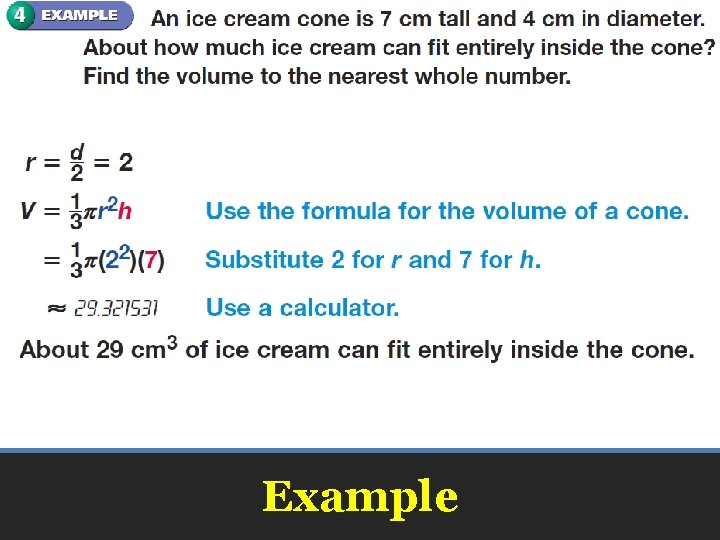ExampleSample Problems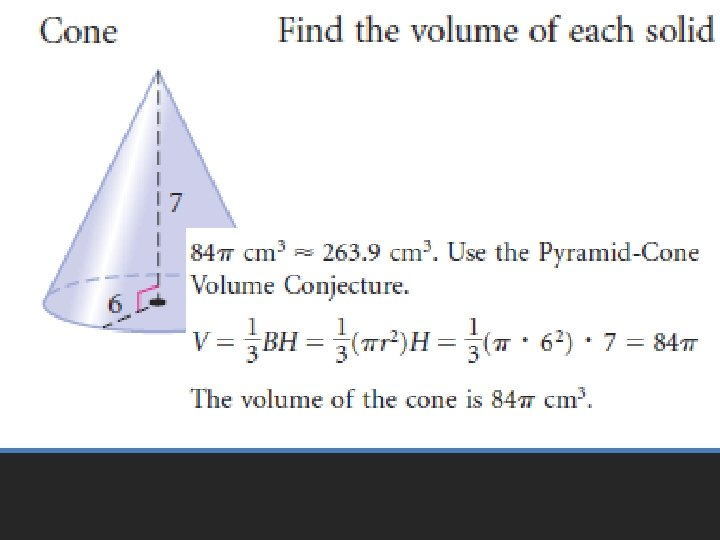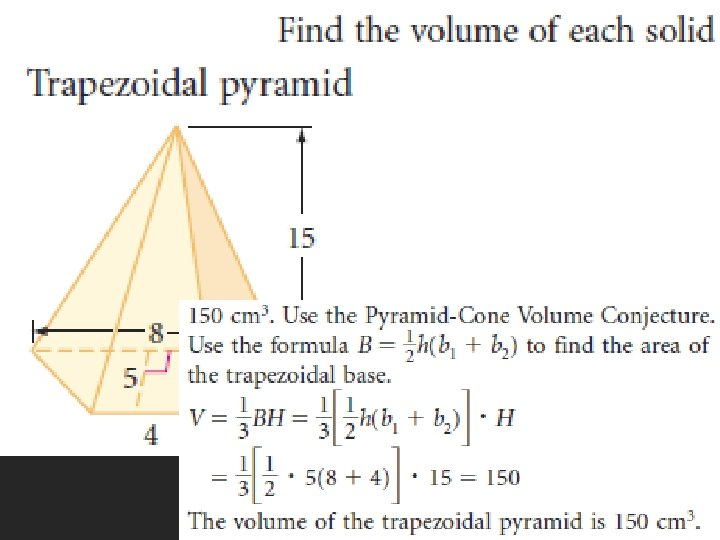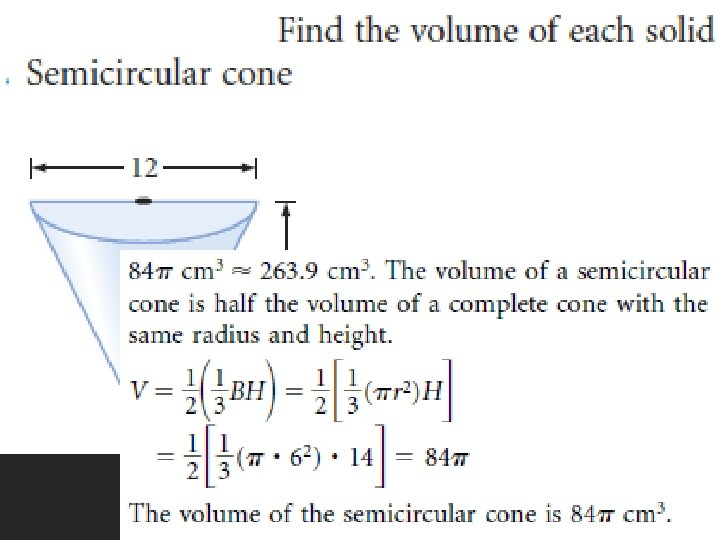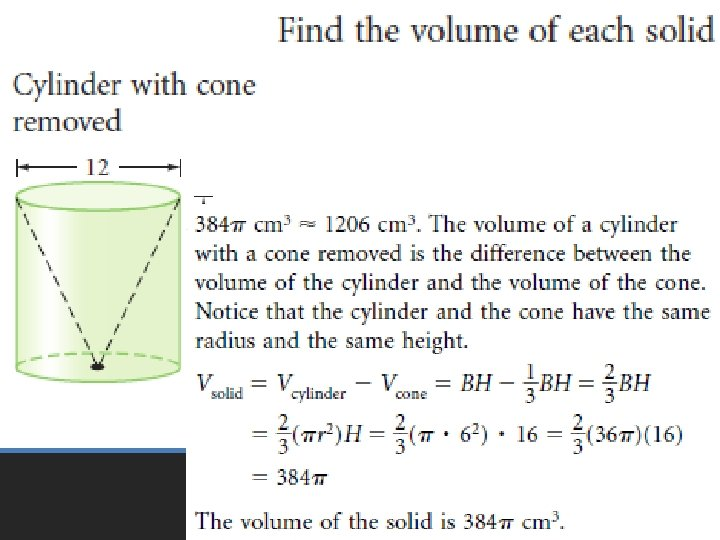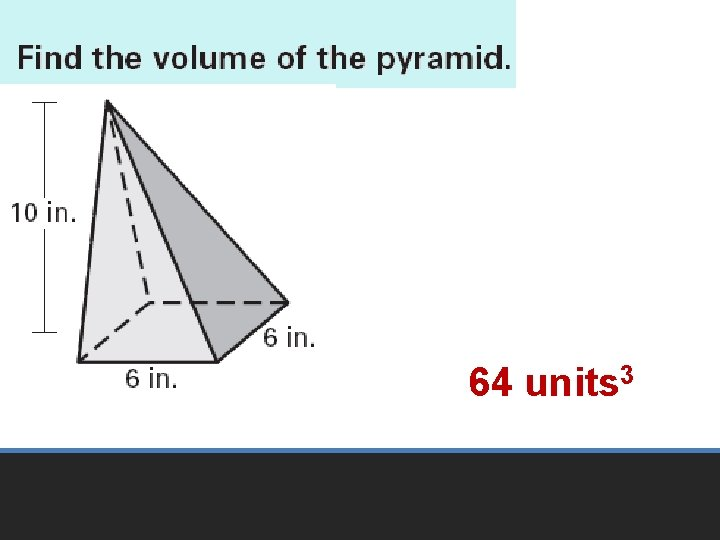64 units 3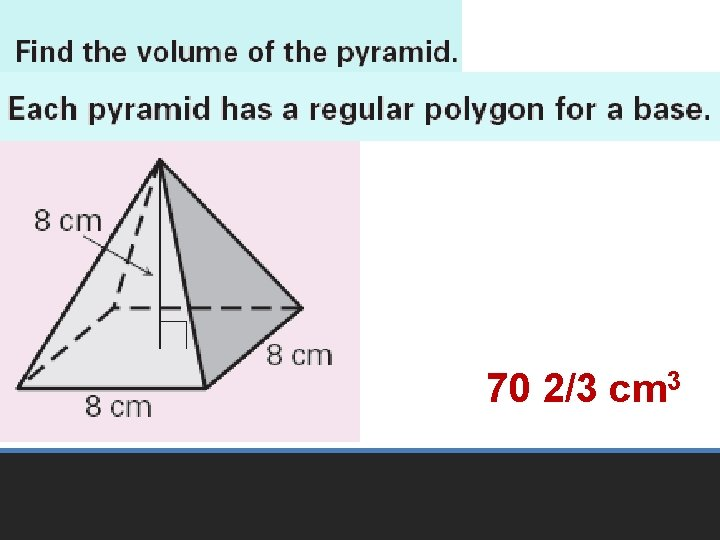70 2/3 cm 3357. 24 in 364 units 370 2/3 cm 3357. 24 in 3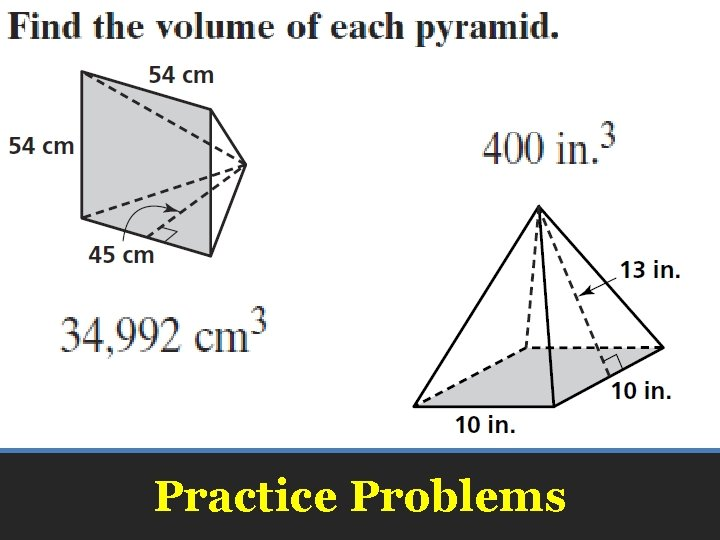Practice ProblemsPractice Problems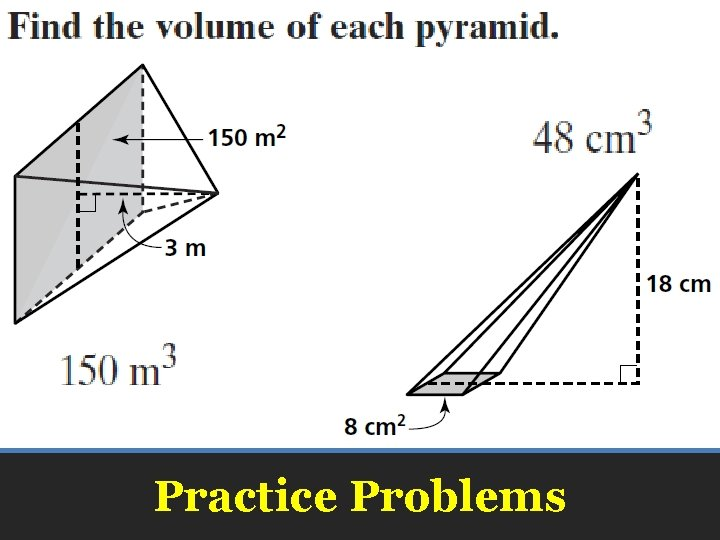Practice Problems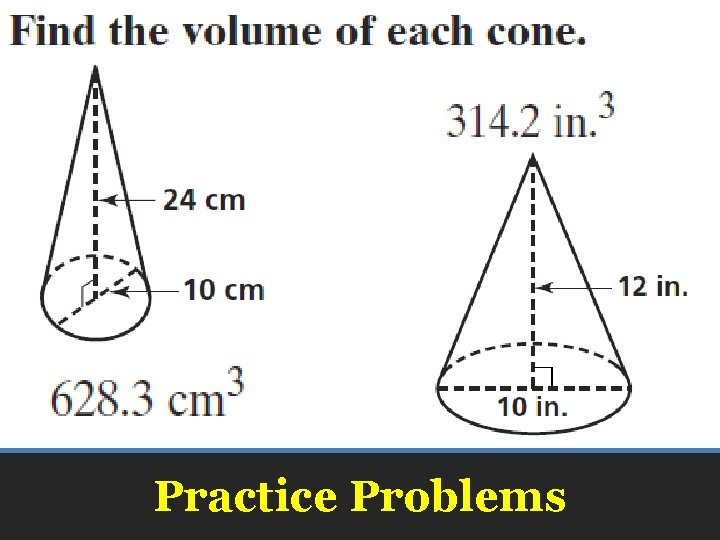Practice Problems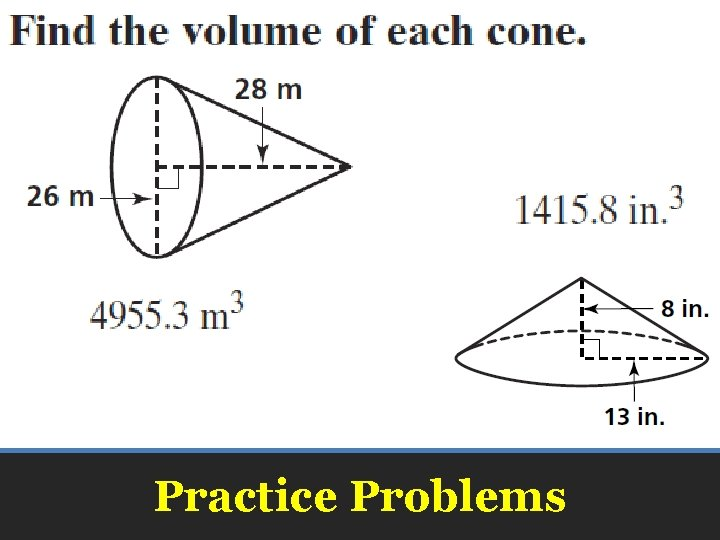Practice Problems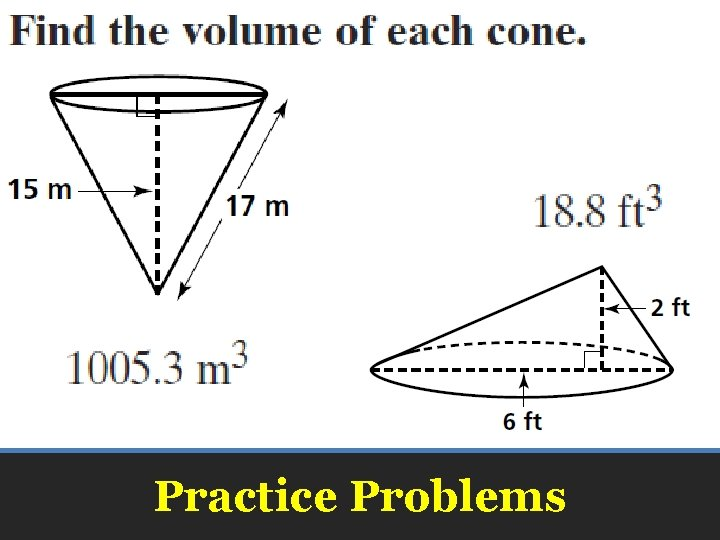Practice ProblemsPractice Problems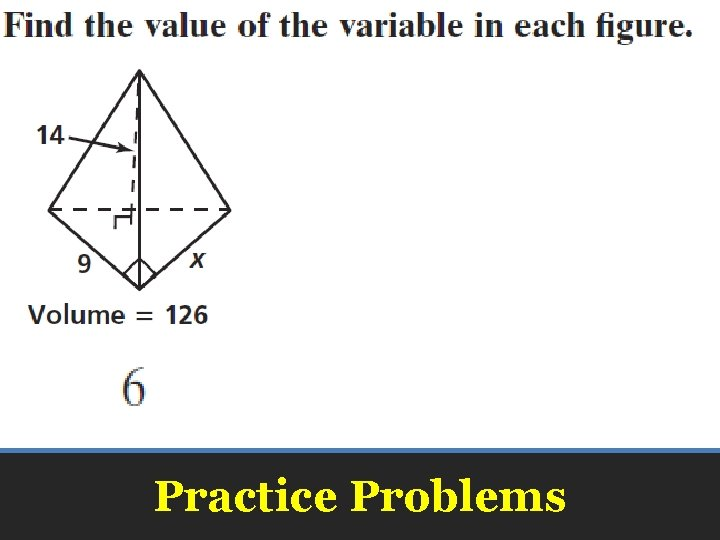Practice Problems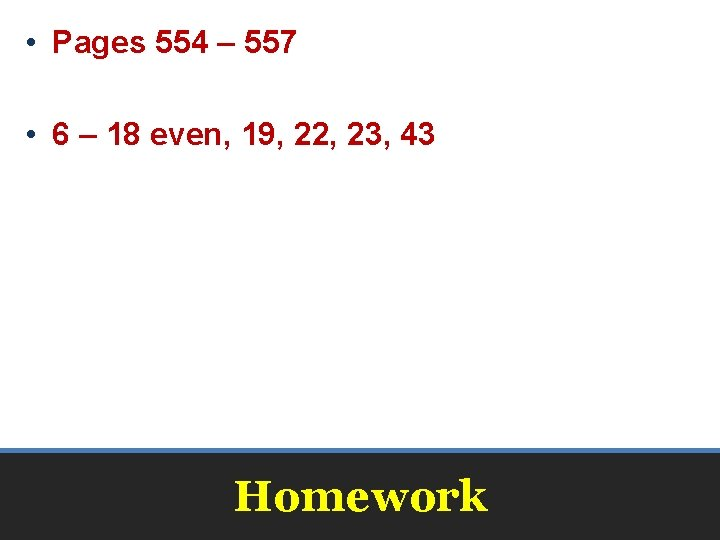• Pages 554 – 557 • 6 – 18 even, 19, 22, 23, 43 Homework• Pages 554 – 557 • 6 – 18 even, 19, 22, 23, 34, 43 Honors Homework